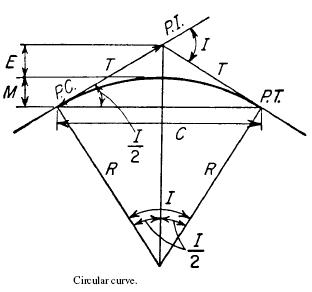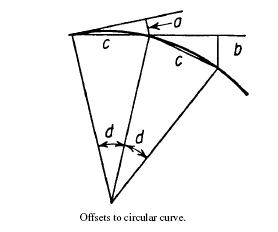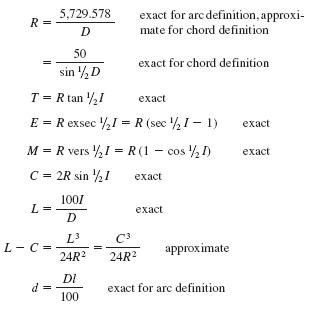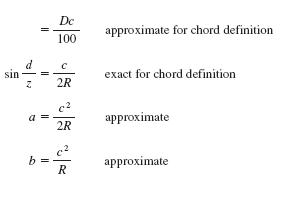Search

# Circular Curves

Posted in Highway Engineering |Email This Post |

The most common type of horizontal curve used to connect intersecting tangent (or straight) sections of highways or railroads are Circular curves. In most countries, two methods of defining circular curves are in use: the first, in general use in railroad work, defines the degree of curve as the central angle subtended by a chord of 100 ft (30.48 m) in length; the second, used in highway work, defines the degree of curve as the central angle subtended by an arc of 100 ft (30.48 m) in length.

The terms and symbols generally used in reference to circular curves are listed next below:PC= point of curvature, beginning of curve
PI= point of intersection of tangents
PT =point of tangency, end of curve
R= radius of curve, ft (m)
D= degree of curve (see previous text)
I= deflection angle between tangents at PI, also central angle of curve
T= tangent distance, distance from PI to PC or PT, ft (m)
L= length of curve from PC to PT measured on 100-ft
(30.48-m) chord for chord definition, on arc for arc definition, ft (m)
C= length of long chord from PC to PT, ft (m)
E= external distance, distance from PI to midpoint of curve, ft (m)

M= midordinate, distance from midpoint of curve to midpoint of long chord, ft (m)
d= central angle for portion of curve (d
l length of curve (arc) determined by central angle
d, ft (m)
c= length of curve (chord) determined by central angle d, ft (m)
a= tangent offset for chord of length c, ft (m)
b= chord offset for chord of length c, ft (m)

Equations of Circular CurvesMore Entries :
• harsh August 3, 2009 at 2:57 pm

i have doubt regarding one structure
why is the shape of roundabouts or traffic circle circular only and not of any other shape i would like to know all the mathematical calculations and exact reason behind it

• TR in Canada September 30, 2010 at 7:14 pm

It’s worked for many years and still will for years to come.

• Lazarus Ighacho November 22, 2010 at 4:01 am

the reason behind it is based on rates of increase of radial acceleration, gravity , speed, sight and vehicular shape. A square shape will be cumbersome or any other shape irregular. However anything with radial aspects works for instance ovular shape or arcs all work as long as the intrinsic characteristics of the road and those of the vehicles work in agreement. that is why circular is the best

• theophilus.nqoko October 15, 2012 at 6:18 am

who first invented the method of circular curve?

• Ranjan Datta April 13, 2017 at 10:18 am

it is useful to find the radius of curve.

• Ranjan Datta April 13, 2017 at 11:20 am

did not know